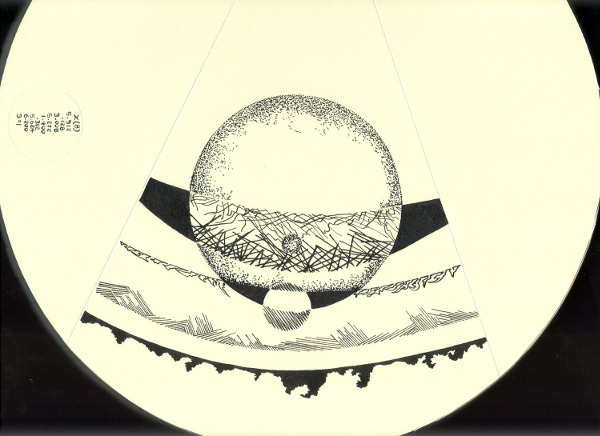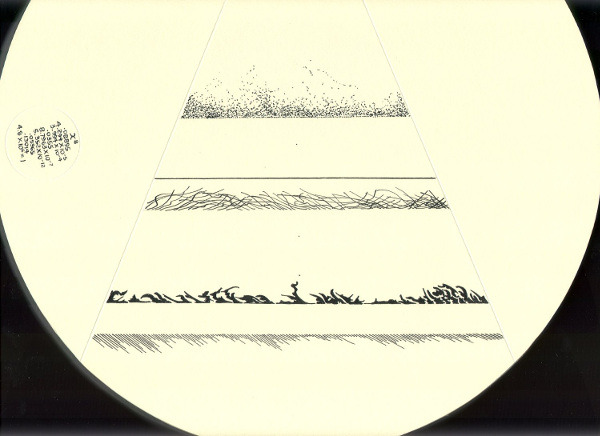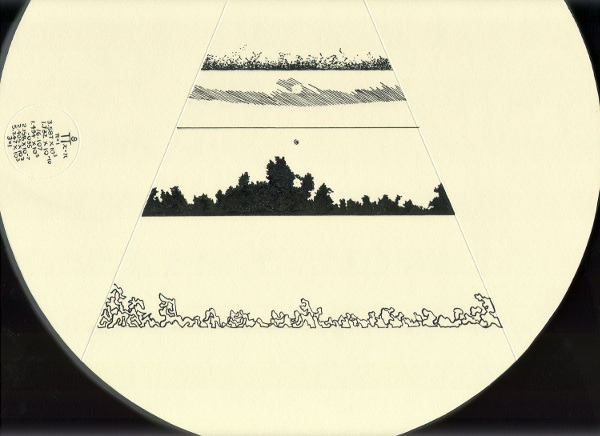## CATALOG NO. 13 1999EightBook fully open

Specifications Unique Book
12" diameter - Eight ink drawings - Plexiglas cover

### Also see Eight - Artist Book Edition

"Eight" is a visual experiment using eight different mathematical operations on the same set of eight random numbers to produce a framework of arcs and circles for eight sets of abstract ink drawings.
The mathematical operations are as follows: sum, difference, product, quotient, raising to the 8th power, taking the 8th root, sum series, and product series. The random numbers, as seen on the title page, are: .739, .016, .376, .659, .175, .039, .703, .775
Each set of drawings has eight elements. Each element is composed of a portion of a circle or arc that has its diameter determined by the result of one of the eight mathematical operations performed on each random number, one mathematical operation per page.
The different mathematical operations produce widely varying results as to scale. Some arcs are almost straight lines and some circles have to be represented as dots. The drawing scale varies from 3 to 1 to more than 4,000,000 to 1.
The order of the random number results listed on each page corresponds to the order of the circle or arc elements, top to bottom. The mathematical operation is depicted at the top of the random number results list and the scale of the arc-circle elements is listed at the bottom. The drawings are pure abstractions drawn along the arc or circle formed by the mathematical operation.Page 1Page 2Page 3Page 4Page 5Page 6Page 7Page 8